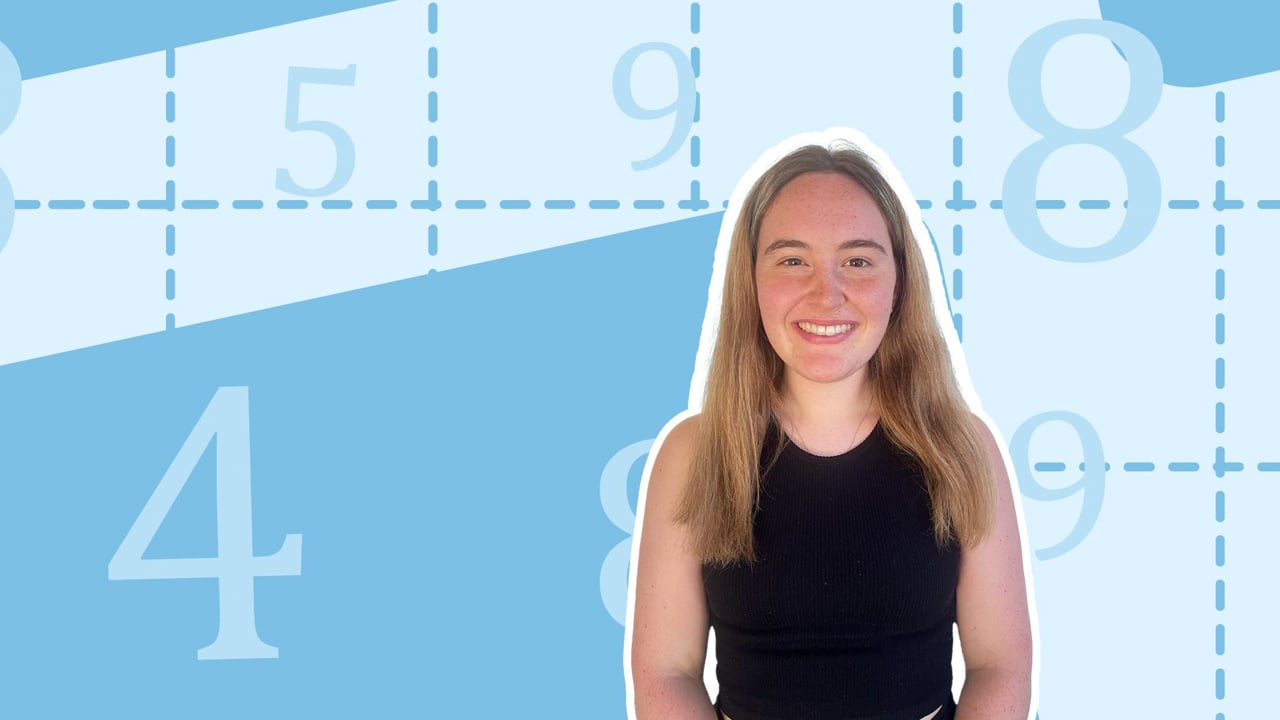Chapter overviewMaths

Exam board

AQA

Number

Algebra

Graphs

Ratio proportion and rates of change

Shapes and area

Angles and geometry

Trigonometry

Probability

Statistics

Maths

# Types of numbers0%

Summary

# Types of numbers

## In a nutshell

Numbers are used to represent quantities of items, and different types of numbers belong to different sets. For example, integers can be used for counting while rational numbers are better used to describe how much of a portion remains.

## Identifying types of numbers

#### Example

Natural
Any positive whole number is a natural number (not including $0$)​
$1, 2,5$​​
Integer
Any whole number is an integer (including $0$)​
$0,7, -3$​​
Rational
Any number that can be expressed as a fraction where in its simplest form both the numerator and denominator are integers
$\dfrac{16}{7}, -\dfrac{1}{3}, 9$​​
Irrational
Any number that cannot be expressed as a fraction
$\pi, \sqrt{2}, \sqrt{\dfrac{17}{3}}$​​
Real
Any number is a real number
$\sqrt{3}, 4, -\dfrac{3}{5}$​​
Prime
Any positive whole number that has only two factors, that being $1$ and itself​
$2,5,13$​​
Square
Any number that can be expressed as the square of a natural number
$1, 4, 16$​​
Cube
Any number that can be expressed as the cube of a natural number
$1, -8,27$​​
Surd
Square rooted numbers and can be positive or negative
$\sqrt{50},\, 3\sqrt46,\, -\sqrt{8}$​​

##### Example 1

What type of number is $8.6$?

$8.6 = \dfrac{86}{10} = \dfrac{43}{5}$

Hence as this is the simplest form and both the numerator and denominator are integers, $8.6$ is a rational number.

## Subsets of numbers

Some of the definitions of numbers overlap. For instance, $6$ falls into the category of a natural number, integer, rational number and also a real number. This gives a subset order for the types of numbers which can be seen below:

From the Venn diagram you can see that all natural numbers are integers, all integers are rational numbers, and all rational and irrational numbers are real.

FAQs

• Question: How do I determine what type a number is?

Answer: Match the number to the definition of the number and then show that the number fits the definition.

• Question: What are the 7 types of numbers?

Answer: Integer, rational, irrational, prime, square, cube, surd.

• Question: What is a rational number?

Answer: Numbers that can be written as fractions, where both the numerator and denominator are integers.

Theory

Exercises